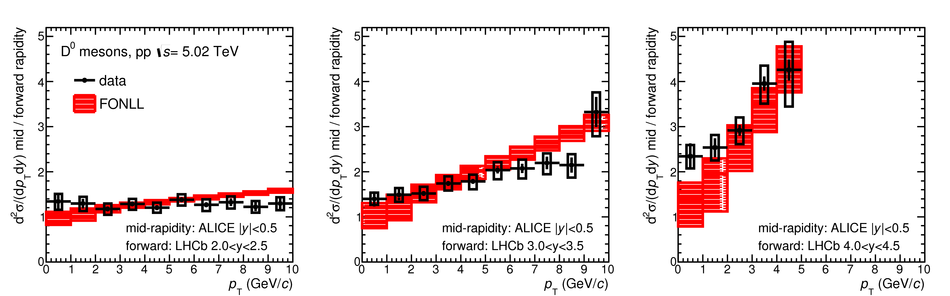# Figure 15

 Ratios of $\Dzero$-meson production cross section per unit of rapidity at mid-rapidity ($|y|< 0.5$) to those measured by the LHCb Collaboration~ in three rapidity ranges, $2< y< 2.5$ (left panel), $3< y< 3.5$ (middle panel), and $4< y< 4.5$ (right panel), as a function of $\pt$. The error bars and boxes represent the statistical and systematic uncertainty, respectively Predictions from FONLL calculations are compared to the data points.